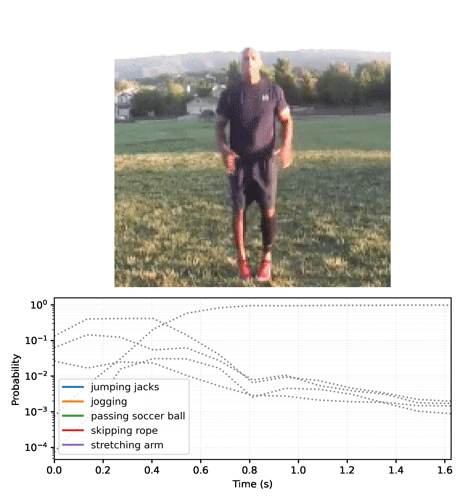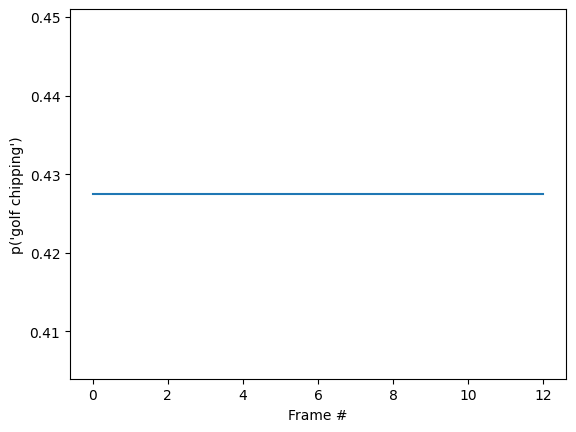Thanks for tuning in to Google I/O. View all sessions on demand

# MoViNet for streaming action recognition

This tutorial demonstrates how to use a pretrained video classification model to classify an activity (such as dancing, swimming, biking etc) in the given video.

The model architecture used in this tutorial is called MoViNet (Mobile Video Networks). MoVieNets are a family of efficient video classification models trained on huge dataset (Kinetics 600).

In contrast to the i3d models available on TF Hub, MoViNets also support frame-by-frame inference on streaming video.

The pretrained models are available from TF Hub. The TF Hub collection also includes quantized models optimized for TFLite.

The source for these models is available in the TensorFlow Model Garden. This includes a longer version of this tutorial that also covers building and fine-tuning a MoViNet model.

This MoViNet tutorial is part of a series of TensorFlow video tutorials. Here are the other three tutorials:## Setup

For inference on smaller models (A0-A2), CPU is sufficient for this Colab.

````sudo apt install -y ffmpeg`
`pip install -q mediapy`
```
````pip uninstall -q -y opencv-python-headless`
`pip install -q "opencv-python-headless<4.3"`
```
``````# Import libraries
import pathlib

import matplotlib as mpl
import matplotlib.pyplot as plt
import mediapy as media
import numpy as np
import PIL

import tensorflow as tf
import tensorflow_hub as hub
import tqdm

mpl.rcParams.update({
'font.size': 10,
})
``````

Get the kinetics 600 label list, and print the first few labels:

``````labels_path = tf.keras.utils.get_file(
fname='labels.txt',
origin='https://raw.githubusercontent.com/tensorflow/models/f8af2291cced43fc9f1d9b41ddbf772ae7b0d7d2/official/projects/movinet/files/kinetics_600_labels.txt'
)
labels_path = pathlib.Path(labels_path)

KINETICS_600_LABELS = np.array([line.strip() for line in lines])
KINETICS_600_LABELS[:20]
``````
```Downloading data from https://raw.githubusercontent.com/tensorflow/models/f8af2291cced43fc9f1d9b41ddbf772ae7b0d7d2/official/projects/movinet/files/kinetics_600_labels.txt
9209/9209 [==============================] - 0s 0us/step
array(['abseiling', 'acting in play', 'adjusting glasses', 'air drumming',
'applying cream', 'archaeological excavation', 'archery',
'arguing', 'arm wrestling', 'arranging flowers',
'assembling bicycle', 'assembling computer',
'attending conference', 'auctioning', 'backflip (human)',
```

To provide a simple example video for classification, we can load a short gif of jumping jacks being performed.``````jumpingjack_url = 'https://github.com/tensorflow/models/raw/f8af2291cced43fc9f1d9b41ddbf772ae7b0d7d2/official/projects/movinet/files/jumpingjack.gif'
jumpingjack_path = tf.keras.utils.get_file(
fname='jumpingjack.gif',
origin=jumpingjack_url,
cache_dir='.', cache_subdir='.',
)
``````
```Downloading data from https://github.com/tensorflow/models/raw/f8af2291cced43fc9f1d9b41ddbf772ae7b0d7d2/official/projects/movinet/files/jumpingjack.gif
783318/783318 [==============================] - 0s 0us/step
```

Define a function to read a gif file into a `tf.Tensor`:

The video's shape is `(frames, height, width, colors)`

``````jumpingjack=load_gif(jumpingjack_path)
jumpingjack.shape
``````
```TensorShape([13, 224, 224, 3])
```

## How to use the model

This section contains a walkthrough showing how to use the models from TensorFlow Hub. If you just want to see the models in action, skip to the next section.

There are two versions of each model: `base` and `streaming`.

• The `base` version takes a video as input, and returns the probabilities averaged over the frames.
• The `streaming` version takes a video frame and an RNN state as input, and returns the predictions for that frame, and the new RNN state.

### The base model

``````%%time
id = 'a2'
mode = 'base'
version = '3'
hub_url = f'https://tfhub.dev/tensorflow/movinet/{id}/{mode}/kinetics-600/classification/{version}'
``````
```CPU times: user 12.3 s, sys: 516 ms, total: 12.8 s
Wall time: 13.8 s
```

This version of the model has one `signature`. It takes an `image` argument which is a `tf.float32` with shape `(batch, frames, height, width, colors)`. It returns a dictionary containing one output: A `tf.float32` tensor of logits with shape `(batch, classes)`.

``````sig = model.signatures['serving_default']
print(sig.pretty_printed_signature())
``````
```signature_wrapper(*, image)
Args:
image: float32 Tensor, shape=(None, None, None, None, 3)
Returns:
<1>: float32 Tensor, shape=(None, 600)
```

To run this signature on the video you need to add the outer `batch` dimension to the video first.

``````#warmup
sig(image = jumpingjack[tf.newaxis, :1]);
``````
``````%%time
logits = sig(image = jumpingjack[tf.newaxis, ...])

print(logits.shape)
print()
``````
```(600,)

CPU times: user 6.98 s, sys: 190 ms, total: 7.17 s
Wall time: 9.19 s
```

Define a `get_top_k` function that packages the above output processing for later.

Convert the `logits` to probabilities, and look up the top 5 classes for the video. The model confirms that the video is probably of `jumping jacks`.

``````probs = tf.nn.softmax(logits, axis=-1)
for label, p in get_top_k(probs):
print(f'{label:20s}: {p:.3f}')
``````
```jumping jacks       : 0.834
zumba               : 0.008
lunge               : 0.003
doing aerobics      : 0.003
polishing metal     : 0.002
```

### The streaming model

The previous section used a model that runs over a whole video. Often when processing a video you don't want a single prediction at the end, you want to update predictions frame by frame. The `stream` versions of the model allow you to do this.

Load the `stream` version of the model.

``````%%time
id = 'a2'
mode = 'stream'
version = '3'
hub_url = f'https://tfhub.dev/tensorflow/movinet/{id}/{mode}/kinetics-600/classification/{version}'
``````
```WARNING:absl:`state/b4/l2/pool_buffer` is not a valid tf.function parameter name. Sanitizing to `state_b4_l2_pool_buffer`.
WARNING:absl:`state/b1/l2/pool_buffer` is not a valid tf.function parameter name. Sanitizing to `state_b1_l2_pool_buffer`.
WARNING:absl:`state/b1/l1/pool_frame_count` is not a valid tf.function parameter name. Sanitizing to `state_b1_l1_pool_frame_count`.
WARNING:absl:`state/b1/l0/pool_frame_count` is not a valid tf.function parameter name. Sanitizing to `state_b1_l0_pool_frame_count`.
WARNING:absl:`state/b2/l2/pool_buffer` is not a valid tf.function parameter name. Sanitizing to `state_b2_l2_pool_buffer`.
CPU times: user 32.7 s, sys: 1.69 s, total: 34.4 s
Wall time: 34.8 s
```

Using this model is slightly more complex than the `base` model. You have to keep track of the internal state of the model's RNNs.

``````list(model.signatures.keys())
``````
```['call', 'init_states']
```

The `init_states` signature takes the video's shape `(batch, frames, height, width, colors)` as input, and returns a large dictionary of tensors containing the initial RNN states:

``````lines = model.signatures['init_states'].pretty_printed_signature().splitlines()
lines = lines[:10]
lines.append('      ...')
print('.\n'.join(lines))
``````
```signature_wrapper(*, input_shape).
Args:.
input_shape: int32 Tensor, shape=(5,).
Returns:.
{'state/b0/l0/pool_buffer': <1>, 'state/b0/l0/pool_frame_count': <2>, 'state/b0/l1/pool_buffer': <3>, 'state/b0/l1/pool_frame_count': <4>, 'state/b0/l1/stream_buffer': <5>, 'state/b0/l2/pool_buffer': <6>, 'state/b0/l2/pool_frame_count': <7>, 'state/b0/l2/stream_buffer': <8>, 'state/b1/l0/pool_buffer': <9>, 'state/b1/l0/pool_frame_count': <10>, 'state/b1/l0/stream_buffer': <11>, 'state/b1/l1/pool_buffer': <12>, 'state/b1/l1/pool_frame_count': <13>, 'state/b1/l1/stream_buffer': <14>, 'state/b1/l2/pool_buffer': <15>, 'state/b1/l2/pool_frame_count': <16>, 'state/b1/l2/stream_buffer': <17>, 'state/b1/l3/pool_buffer': <18>, 'state/b1/l3/pool_frame_count': <19>, 'state/b1/l3/stream_buffer': <20>, 'state/b1/l4/pool_buffer': <21>, 'state/b1/l4/pool_frame_count': <22>, 'state/b1/l4/stream_buffer': <23>, 'state/b2/l0/pool_buffer': <24>, 'state/b2/l0/pool_frame_count': <25>, 'state/b2/l0/stream_buffer': <26>, 'state/b2/l1/pool_buffer': <27>, 'state/b2/l1/pool_frame_count': <28>, 'state/b2/l1/stream_buffer': <29>, 'state/b2/l2/pool_buffer': <30>, 'state/b2/l2/pool_frame_count': <31>, 'state/b2/l2/stream_buffer': <32>, 'state/b2/l3/pool_buffer': <33>, 'state/b2/l3/pool_frame_count': <34>, 'state/b2/l3/stream_buffer': <35>, 'state/b2/l4/pool_buffer': <36>, 'state/b2/l4/pool_frame_count': <37>, 'state/b2/l4/stream_buffer': <38>, 'state/b3/l0/pool_buffer': <39>, 'state/b3/l0/pool_frame_count': <40>, 'state/b3/l0/stream_buffer': <41>, 'state/b3/l1/pool_buffer': <42>, 'state/b3/l1/pool_frame_count': <43>, 'state/b3/l1/stream_buffer': <44>, 'state/b3/l2/pool_buffer': <45>, 'state/b3/l2/pool_frame_count': <46>, 'state/b3/l2/stream_buffer': <47>, 'state/b3/l3/pool_buffer': <48>, 'state/b3/l3/pool_frame_count': <49>, 'state/b3/l3/stream_buffer': <50>, 'state/b3/l4/pool_buffer': <51>, 'state/b3/l4/pool_frame_count': <52>, 'state/b3/l5/pool_buffer': <53>, 'state/b3/l5/pool_frame_count': <54>, 'state/b3/l5/stream_buffer': <55>, 'state/b4/l0/pool_buffer': <56>, 'state/b4/l0/pool_frame_count': <57>, 'state/b4/l0/stream_buffer': <58>, 'state/b4/l1/pool_buffer': <59>, 'state/b4/l1/pool_frame_count': <60>, 'state/b4/l2/pool_buffer': <61>, 'state/b4/l2/pool_frame_count': <62>, 'state/b4/l3/pool_buffer': <63>, 'state/b4/l3/pool_frame_count': <64>, 'state/b4/l4/pool_buffer': <65>, 'state/b4/l4/pool_frame_count': <66>, 'state/b4/l5/pool_buffer': <67>, 'state/b4/l5/pool_frame_count': <68>, 'state/b4/l5/stream_buffer': <69>, 'state/b4/l6/pool_buffer': <70>, 'state/b4/l6/pool_frame_count': <71>, 'state/head/pool_buffer': <72>, 'state/head/pool_frame_count': <73>}.
<1>: float32 Tensor, shape=(None, 1, 1, 1, 40).
<2>: int32 Tensor, shape=(1,).
<3>: float32 Tensor, shape=(None, 1, 1, 1, 40).
<4>: int32 Tensor, shape=(1,).
<5>: float32 Tensor, shape=(None, 2, None, None, 40).
...
```
``````initial_state = model.init_states(jumpingjack[tf.newaxis, ...].shape)
``````
``````type(initial_state)
``````
```dict
```
``````list(sorted(initial_state.keys()))[:5]
``````
```['state/b0/l0/pool_buffer',
'state/b0/l0/pool_frame_count',
'state/b0/l1/pool_buffer',
'state/b0/l1/pool_frame_count',
'state/b0/l1/stream_buffer']
```

Once you have the initial state for the RNNs, you can pass the state and a video frame as input (keeping the `(batch, frames, height, width, colors)` shape for the video frame). The model returns a `(logits, state)` pair.

After just seeing the first frame, the model is not convinced that the video is of "jumping jacks":

``````inputs = initial_state.copy()

# Add the batch axis, take the first frme, but keep the frame-axis.
inputs['image'] = jumpingjack[tf.newaxis, 0:1, ...]
``````
``````# warmup
model(inputs);
``````
``````logits, new_state = model(inputs)
logits = logits
probs = tf.nn.softmax(logits, axis=-1)

for label, p in get_top_k(probs):
print(f'{label:20s}: {p:.3f}')

print()
``````
```golf chipping       : 0.427
tackling            : 0.134
lunge               : 0.056
stretching arm      : 0.053
passing american football (not in game): 0.039
```

If you run the model in a loop, passing the updated state with each frame, the model quickly converges to the correct result:

``````%%time
state = initial_state.copy()
all_logits = []

for n in range(len(jumpingjack)):
inputs = state
inputs['image'] = jumpingjack[tf.newaxis, n:n+1, ...]
result, state = model(inputs)
all_logits.append(logits)

probabilities = tf.nn.softmax(all_logits, axis=-1)
``````
```CPU times: user 371 ms, sys: 14.2 ms, total: 385 ms
Wall time: 356 ms
```
``````for label, p in get_top_k(probabilities[-1]):
print(f'{label:20s}: {p:.3f}')
``````
```golf chipping       : 0.427
tackling            : 0.134
lunge               : 0.056
stretching arm      : 0.053
passing american football (not in game): 0.039
```
``````id = tf.argmax(probabilities[-1])
plt.plot(probabilities[:, id])
plt.xlabel('Frame #')
plt.ylabel(f"p('{KINETICS_600_LABELS[id]}')");
``````You may notice that the final probability is much more certain than in the previous section where you ran the `base` model. The `base` model returns an average of the predictions over the frames.

``````for label, p in get_top_k(tf.reduce_mean(probabilities, axis=0)):
print(f'{label:20s}: {p:.3f}')
``````
```golf chipping       : 0.427
tackling            : 0.134
lunge               : 0.056
stretching arm      : 0.053
passing american football (not in game): 0.039
```

## Animate the predictions over time

The previous section went into some details about how to use these models. This section builds on top of that to produce some nice inference animations.

The hidden cell below to defines helper functions used in this section.

Start by running the streaming model across the frames of the video, and collecting the logits:

``````init_states = model.init_states(jumpingjack[tf.newaxis].shape)
``````
``````# Insert your video clip here
video = jumpingjack
images = tf.split(video[tf.newaxis], video.shape, axis=1)

all_logits = []

# To run on a video, pass in one frame at a time
states = init_states
for image in tqdm.tqdm(images):
# predictions for each frame
logits, states = model({**states, 'image': image})
all_logits.append(logits)

# concatinating all the logits
logits = tf.concat(all_logits, 0)
# estimating probabilities
probs = tf.nn.softmax(logits, axis=-1)
``````
```100%|██████████| 13/13 [00:00<00:00, 36.41it/s]
```
``````final_probs = probs[-1]
print('Top_k predictions and their probablities\n')
for label, p in get_top_k(final_probs):
print(f'{label:20s}: {p:.3f}')
``````
```Top_k predictions and their probablities

jumping jacks       : 0.999
zumba               : 0.000
doing aerobics      : 0.000
dancing charleston  : 0.000
slacklining         : 0.000
```

Convert the sequence of probabilities into a video:

``````# Generate a plot and output to a video tensor
plot_video = plot_streaming_top_preds(probs, video, video_fps=8.)
``````
```100%|██████████| 13/13 [00:06<00:00,  1.99it/s]
```
``````# For gif format, set codec='gif'
media.show_video(plot_video, fps=3)
``````

## Resources

The pretrained models are available from TF Hub. The TF Hub collection also includes quantized models optimized for TFLite.

The source for these models is available in the TensorFlow Model Garden. This includes a longer version of this tutorial that also covers building and fine-tuning a MoViNet model.